# Check If a Value Exists Using VLOOKUP Formula

Generic Formula:

 =IF(ISERROR(VLOOKUP(value,range,column number,0)),"No","Yes")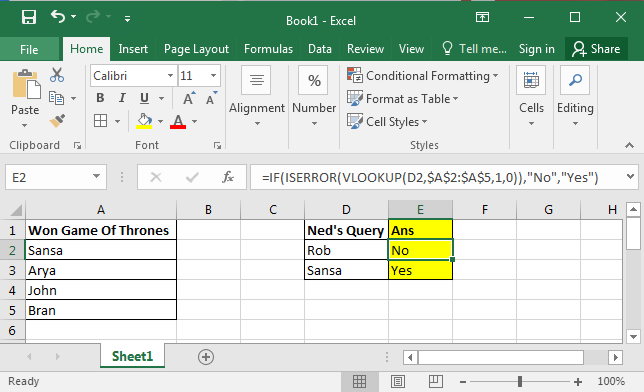The Excel VLOOKUP function is the most frequently used function in excel and it is mostly used to return value if value is in range . One can not work effectively without VLOOKUP on Microsoft Excel.

The basic use of VLOOKUP is to retrieve data from one range/sheet/workbook to another, based on some unique ID or value. But using VLOOKUP we can do many other tasks.

In this tutorial we will learn how to check if a given value exists in a list or not , using VLOOKUP.

## VLOOKUP Example:

Let's say, Ned Stark wants to know if his child Rob Stark has won the GAME OF THRONES or not.

So you have a list of characters who won (survived) this Game of Thrones. We have Ned’s query in column D.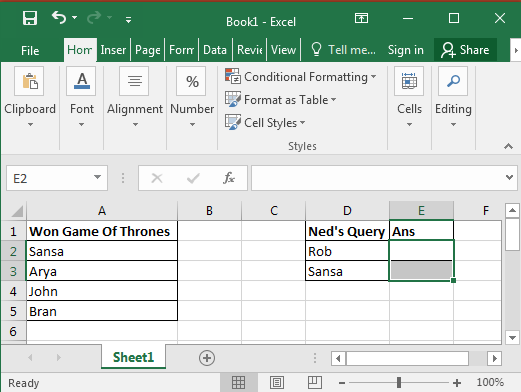Now we need to check the values in the list to see if they exist or not using VLOOKUP.

Generic VLOOKUP Formula Syntax

 =VLOOKUP(value to check, list range, column number, 0/1)

or

 =VLOOKUP(lookup_value, table_array, col_index_num, [lookup_range]) :excel syntax

Value to check: The first argument is the value you want to find. We want to look for Rob and Sansa,

List Range: This is your list from where excel lookup values will be found. Here it is in Range A2:A5 (we are taking a small list for better understanding. It can be lakh rows and thousands of columns)

Column Number: This is the column number from where you want to fetch value in your range. Since our range is only in A column, it is 1 for us.

0/1: The last argument is very important. If you want to find an exact match, give 0 or FALSE, and if you want to find the nearest value or say approximate then give 1 or TRUE. We want to find Exact Match so we will give 0 as an argument.

Let’s bring it together in Cell E2 and write this VLOOKUP Formula:

 =VLOOKUP(D2,\$A\$2:\$A\$5,1,0)

Copy this formula from E3. You will see a similar image below in your excel: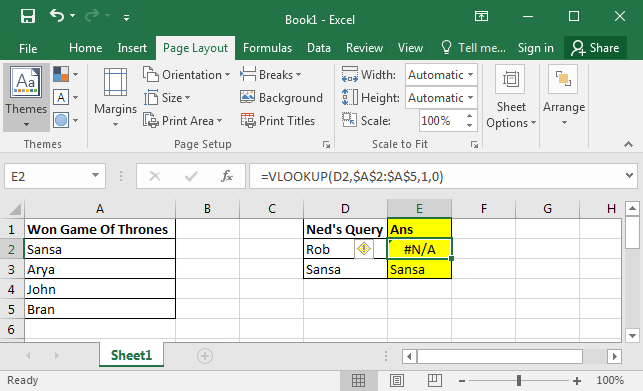VLOOKUP looks for the supplied lookup value in the given range. If the value is not found it returns an error #N/A. If value is found, excel returns the value.

Hence Rob is not on the list and Sansa is there. But you probably won't want to send this kind of report. You want to send if “Yes” if he won and “NO” if not.

To do that we use IF and ISERROR.

ISERROR simply checks to see if the formula is returning an error or not. If there is an error it Returns TRUE  else FALSE.

It takes only one argument. You can supply anything but most of the time we send it a formula to validate.

When you update the formula below in Cell E2 and copy it into E3. You will have this.

 =ISERROR(VLOOKUP(D2,\$A\$2:\$A\$5,1,0))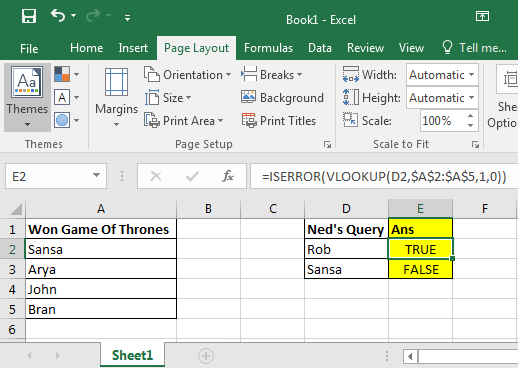Now based on the value returned byISERROR, we can use IF here to get desired results.

If there is an error then “No” else “Yes” they won.

Write this formula in Cell in E2:

 =IF(ISERROR(VLOOKUP(D2,\$A\$2:\$A\$5,1,0)),"NO","YES")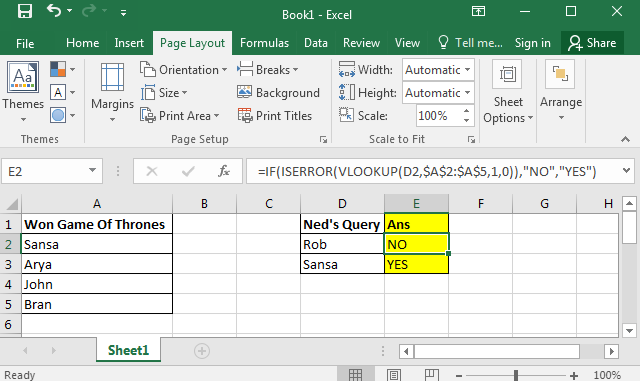Here we learned how to use VLOOKUP formula in excel to find if a value is in a list or not. We had both data in same sheet. Excel lookups value in the another sheet too. You just need to give sheet name before range to excel lookup value in another sheet Even excel can VLOOKUP from different workbooks. The process is same as this. These formulas are available in Excel 2016, 2013, 2010 and in some older versions of excel too.

Related Articles:

Check If Value Is In List in Excel

Check If value is between the two numbers

Check If Cell Contains Specific Text

Partial match with VLOOKUP function

Popular Articles:

50 Excel Shortcut to Increase Your Productivity

The VLOOKUP Function in Excel

COUNTIF in Excel 2016

How to Use SUMIF Function in Excel

1.